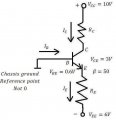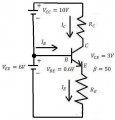# Bjt transistor resistor

#### helloeveryone

Joined Apr 8, 2011
64
I would appreciate it if someone could help me solve the following problem:

Find R_E step by step.

NPN BJT
Positive V_CC=10V
R_Collector=present, value not provided
R_Emitter=present, value not provided
Negative V_EE=6V
V_BE=0.6V
V_CE=3V
ß=50
Base connected to reference point, chassis ground, no resistor present at base.

Work:
V_CC+V_EE=V_RC+V_CE+V_RE
V_CC+V_EE=R_C*I_C+V_CE+R_E*I_E
V_CC+V_EE=R_C*I_C+V_CE+R_E(I_C+I_B)

I_C=ß*I_B

V_EE=V_BE+V_RE
V_EE=V_BE+R_E*I_E
V_EE=V_BE+R_E(I_C+I_B)
V_EE=V_BE+R_E(I_C+I_C/ß)
V_EE=V_BE+R_E*I_C(1+1/ß)

How does one find I_C and R_E from this?Last edited:

#### WBahn

Joined Mar 31, 2012
26,492
Gee, it would sure help if you actually provided a schematic of the circuit you are talking about.

#### helloeveryone

Joined Apr 8, 2011
64
Gee, it would sure help if you actually provided a schematic of the circuit you are talking about.
Better?

#### WBahn

Joined Mar 31, 2012
26,492
Okay.

So what is it you are being asked to find?

#### shteii01

Joined Feb 19, 2010
4,644
So.
How many unknowns do you have?

#### helloeveryone

Joined Apr 8, 2011
64
Okay.

So what is it you are being asked to find?
The problem asks us to find the value of the resistor R_E in ohms. However, two answers are provided R_E and I_C. That makes me think that we need to find I_C first so that we can find R_E.

#### WBahn

Joined Mar 31, 2012
26,492
The problem asks us to find the value of the resistor R_E in ohms. However, two answers are provided R_E and I_C. That makes me think that we need to find I_C first so that we can find R_E.
But how can you find I_C?

The problem doesn't appear to be adequately constrained since you are given neither I_C or I_E nor R_C. So you could throw a dart and randomly pick a value of R_E and, from that, find a value of R_C that yields a V_CE of 3 V.

What, exactly, does the entire problem statement say?

#### shteii01

Joined Feb 19, 2010
4,644
The problem asks us to find the value of the resistor R_E in ohms. However, two answers are provided R_E and I_C. That makes me think that we need to find I_C first so that we can find R_E.
One of the possible shortcuts is to make Ic==Ie. The justification for this action is that Ie=Ic+Ib, but Ib is very small and has very little effect. So, if the user does not need an answer that is correct to 6 decimal places, then the user can make an assumption that Ic=Ie. This way you remove one unknown and you will need one less equation to solve the whole thing.

#### helloeveryone

Joined Apr 8, 2011
64
The problem: Find the resistance R_E in the circuit if V_CE=3V, V_BE=0.6V, and the current gain factor is ß=50.
I_C=0.00019 A
R_E=2790 Ω

#### WBahn

Joined Mar 31, 2012
26,492
The problem: Find the resistance R_E in the circuit if V_CE=3V, V_BE=0.6V, and the current gain factor is ß=50.
I_C=0.00019 A
R_E=2790 Ω
The problem, as stated, is underdetermined. The best you can do is come up with a ratio of R_C to R_E.

We can ask if their provided answer makes sense. They claim R_E is 2790 Ω. Okay, so given that circuit, how much current is flowing in R_E? Is that in any way compatible with the claim that I_C is only 0.19 mA?

#### WBahn

Joined Mar 31, 2012
26,492
One of the possible shortcuts is to make Ic==Ie. The justification for this action is that Ie=Ic+Ib, but Ib is very small and has very little effect. So, if the user does not need an answer that is correct to 6 decimal places, then the user can make an assumption that Ic=Ie. This way you remove one unknown and you will need one less equation to solve the whole thing.
With a beta of 50, making the assumption that Ic = Ie puts you in the range of not even two sig figs.

But even if you are willing to accept that (which is at least reasonable to get a ballpark answer), there still doesn't seem to be enough information to solve the problem.

Example: Randomly pick R_C to be 1 kΩ.

From the problem statement we know what the collector voltage is and so we can find I_C, the collector current (the current in R_C). We know beta so we can find I_E. We know the voltage across R_E and so now, with the current through it, we can find R_E.

Now randomly pick R_C to be 10 kΩ and repeat the process.

You get a different pair of values for I_C and R_E. Both satisfy the problem constraints.

There are infinitely many solutions.

What might be interesting is to plot I_C versus R_E.

#### helloeveryone

Joined Apr 8, 2011
64
Does anything change, in terms of making the problem solvable, if the reference voltage symbol is changed to ground, ie. 0 Volts?

#### WBahn

Joined Mar 31, 2012
26,492
The pic says that Chassis not zero. How many volts at the chassis?
I have no idea what to make of that "not 0" comment. I think the person that came up with the problem needs to repeat this course themselves.

#### shteii01

Joined Feb 19, 2010
4,644
Does anything change, in terms of making the problem solvable, if the reference voltage symbol is changed to ground, ie. 0 Volts?
Not really. You have 3 or 4 unknowns, and two equations, that is not enough equations to find all the unknowns.

#### WBahn

Joined Mar 31, 2012
26,492
Does anything change, in terms of making the problem solvable, if the reference voltage symbol is changed to ground, ie. 0 Volts?
It's hard to say because it's unclear what they mean by "not 0" in the first place.

But even if we assume that the chassis ground is at the same reference potential use for V_CC and V_EE, that doesn't address the apparent fact that the problem is undetermined.

#### helloeveryone

Joined Apr 8, 2011
64
I have no idea what to make of that "not 0" comment. I think the person that came up with the problem needs to repeat this course themselves.
I'm new to this stuff I think the straight line symbol which I assumed was chassis ground is actually earth ground, so it could actually be 0. Why can't it just be the positve side of V_EE so +6Volts? Is this what the actual schematic would look like with batteries? Maybe I'm just missing a ground at the dot.Last edited:

#### WBahn

Joined Mar 31, 2012
26,492
I'm new to this stuff I think the straight line symbol which I assumed was chassis ground is actually earth ground, so it could actually be 0. Why can't it just be the positve side of V_EE so +6Volts? Is this what the actual schematic would look like with batteries? Maybe I'm just missing a ground at the dot.
View attachment 102861
That's about the only reasonable thing you can assume based on the information we've been given.

Note that if it is the positive side of V_EE, then it is at 0V, not +6 V. V_EE is specified as being at -6 V. So something that is 6 V higher than that would be at 0 V.

#### helloeveryone

Joined Apr 8, 2011
64
Oh, I see. What about this... let's say they just forgot to assign R_C, using the provided current I_C, I=V/R, R=V/I so R_C=10V/0.00019 A =5263 Ω. Now how does that change the problem if R_C is assigned at R_C= 5263 Ω.

#### JoeJester

Joined Apr 26, 2005
4,390
Maybe the constraint is scientific notation using two decimal places.

The answer could be as small as 9.99 x 10 to the minus 99.

#### WBahn

Joined Mar 31, 2012
26,492
Oh, I see. What about this... let's say they just forgot to assign R_C, using the provided current I_C, I=V/R, R=V/I so R_C=10V/0.00019 A =5263 Ω.
You are making one of the classic Ohm's Law mistakes. You are grabbing the nearest V and the nearest I and throwing them at Ohm's Law just because you want to get an R.

Ohm's Law relates the resistance of a resistor to the voltage ACROSS THAT resistor and the current THROUGH THAT resistor. Is 10 V the voltage drop across R_C?

Now how does that change the problem if R_C is assigned at R_C= 5263 Ω.[/QUOTE]

It makes it solvable. But if had answered the question I asked Post #10, you would already know that the two answers they give are not compatible with each other.